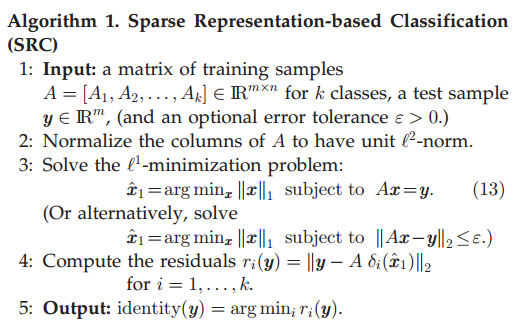# 稀疏表示人脸识别入门文章-《Robust Face Recognition via Sparse Representation》马毅

人脸识别是机器视觉中非常火的一个领域了，在近十几年来发展迅猛，而本文介绍的方法是基于稀疏表示分类（Sparse Representation based classification，SRC）的人脸识别，稀疏表示理论非常强大，突破了传统采样定了的束缚，信号的稀疏表示即用尽可能少的非零元素表示信号主要信息，简化信号处理的求解过程，马毅在09年将稀疏表示引入人脸识别中，其性能相比之前的人脸识别方法具有突破性的进展。

相信很多同学都听说过NN和NS两种分类器，NN对与测试样本的分类是根据单个训练样本与测试样本之间的表示，而NS则是根据每类的训练样本对测试样本的线性表示进行分类识别，SRC则是介于这两种分类器之间的一种人脸分类器，选用部分训练样本对测试样本进行紧凑表示。

接下来对SRC主要步骤进行阐述：在进行稀疏表示人脸分类之前，对训练样本和测试样本进行预处理，即对齐、剪切、归一化，假设有k类带标签的样本，将每张图片按列进行排放， Ai=[vi,1,vi,2,...,vi,ni] $\mathbf{A}_{i}=\left [ v_{i,1},v_{i,2},...,v_{i,n_{i}} \right ]$,根据子空间理论，来自同一样本的样本位于相同的线性子空间中，故假设第 ith $\mathit{i}th$训练样本数目充足，来自第 ith $\mathit{i}th$的测试样本 y $\mathit{y}$可表示为：
y=αi,1vi,1+αi,2vi,2+...+αi,nivi,ni $\mathbf{\mathit{y}}=\alpha _{i,1}v_{i,1}+\alpha _{i,2}v_{i,2}+...+\alpha _{i,n_{i}}v_{i,n_{i}}$

A=[A1,A2,...,Ak]=[v1,1,v1,2,...,vk,nk]$A=[A_{1},A_{2},...,A_{k}]=[v_{1,1},v_{1,2},...,v_{k,n_{k}}]$

y=Ax0 $\mathbf{y}=Ax_{0}$

接下来讨论 y=Ax $\mathbf{y}=Ax$的求解问题，求解向量的稀疏解，可以转化成为下面优化问题：
x^0=argminx0 $\hat{x}_{_{0}}=\arg min\left \| x \right \|_0$ s.t. $s.t.$ Ax=y $Ax=y$,但是在求解 l0norm $l_{0}-norm$时是个NP-Hard问题（优化问题具体我也不懂，反正就是不好正面求解，既然正着不好求，那就想别的办法，这里用近似求解），此处转化成 l1norm $l_{1}-norm$求解问题：
x^1=argminx1 $\hat{x}_{_{1}}=\arg min\left \| x \right \|_1$ s.t. $s.t.$ Ax=y $Ax=y$
（论文中有解释，几何意义上的，数学不好，不能分析了，自行看吧。马毅的主页还有很多关于优化问题的文献）作者针对传统人脸识别过程中特征提取方式和样本处于遮挡进行分析，对于特征提取方式对SRC的影响，在大于120D时各种方法差别不大，所以通常的特征提取方法对于分类系统没有影响；对于遮挡问题，本文采用在字典加入单位矩阵作为误差矩阵，消除遮挡或噪声干扰影响，实验非常丰富，总而言之证明，SRC在对于普通遮挡效果还是行的（但是效果提高空间巨大，所以这是做稀疏人脸识别的改进点之一）。
PS：对于认证问题，本文提出SCI，可以判定是否为数据库中的图片或者非人脸，残差进行分类，稀疏系数进行认证判别，提高识别率；对于表情识别也是有效的。

03-212万+11-19
03-11
06-151537
04-082395
05-12835
08-171568
10-19